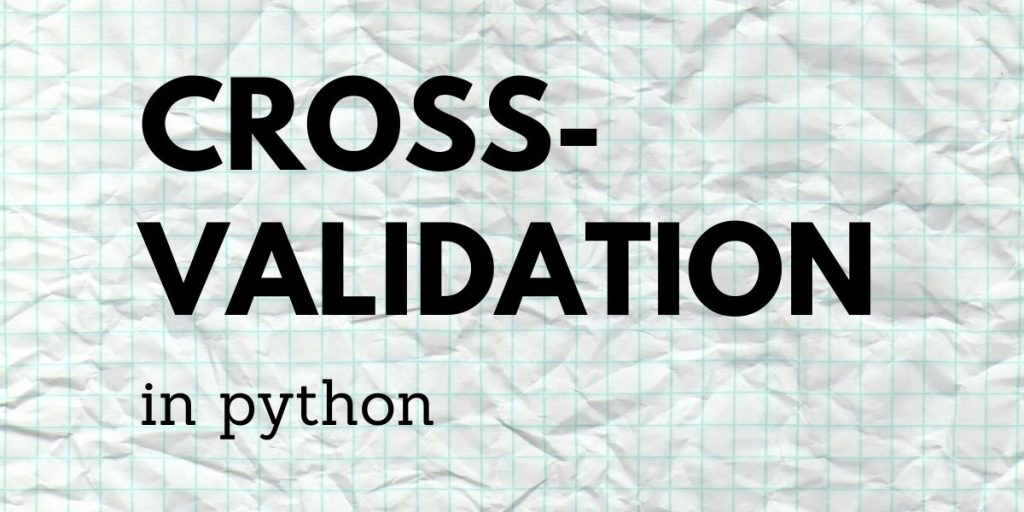# K-Fold Cross-Validation in Python Using SKLearnSplitting a dataset into training and testing set is an essential and basic task when comes to getting a machine learning model ready for training. To determine if our model is overfitting or not we need to test it on unseen data (Validation set).

If a given model does not perform well on the validation set then it’s gonna perform worse when dealing with real live data. This notion makes Cross-Validation probably one of the most important concepts of machine learning which ensures the stability of our model.

Cross-Validation is just a method that simply reserves a part of data from the dataset and uses it for testing the model(Validation set), and the remaining data other than the reserved one is used to train the model.

In this article, we’ll implement cross-validation as provided by sci-kit learn. We’ll implement K-Fold Cross-validation.

## Cross-Validation Intuition

Let’s first see why we should use cross validation.

• It helps us with model evaluation finally determining the quality of the model.
• Crucial to determining if the model is generalizing well to data.
• To check if the model is overfitting or underfitting.
• Finally, it lets us choose the model which had the best performance.

There are many types of Cross Validation Techniques:

• Leave one out cross validation
• k-fold cross validation
• Stratified k-fold cross validation
• Time Series cross validation

## Implementing the K-Fold Cross-Validation

The dataset is split into ‘k’ number of subsets, k-1 subsets then are used to train the model and the last subset is kept as a validation set to test the model. Then the score of the model on each fold is averaged to evaluate the performance of the model.

## K-fold Cross Validation using scikit learn

```#Importing required libraries
import pandas as pd
from sklearn.model_selection import KFold
from sklearn.linear_model import LogisticRegression
from sklearn.metrics import accuracy_score

df = data.frame
X = df.iloc[:,:-1]
y = df.iloc[:,-1]

#Implementing cross validation

k = 5
kf = KFold(n_splits=k, random_state=None)
model = LogisticRegression(solver= 'liblinear')

acc_score = []

for train_index , test_index in kf.split(X):
X_train , X_test = X.iloc[train_index,:],X.iloc[test_index,:]
y_train , y_test = y[train_index] , y[test_index]

model.fit(X_train,y_train)
pred_values = model.predict(X_test)

acc = accuracy_score(pred_values , y_test)
acc_score.append(acc)

avg_acc_score = sum(acc_score)/k

print('accuracy of each fold - {}'.format(acc_score))
print('Avg accuracy : {}'.format(avg_acc_score))
```
```accuracy of each fold - [0.9122807017543859, 0.9473684210526315, 0.9736842105263158, 0.9736842105263158, 0.9557522123893806]
Avg accuracy : 0.952553951249806
```

In the code above we implemented 5 fold cross-validation.

sklearn.model_selection module provides us with KFold class which makes it easier to implement cross-validation. `KFold` class has `split` method which requires a dataset to perform cross-validation on as an input argument.

We performed a binary classification using Logistic regression as our model and cross-validated it using 5-Fold cross-validation. The average accuracy of our model was approximately 95.25%

Feel free to check Sklearn KFold documentation here.

## Cross Validation Using cross_val_score()

You can shorten the above code using `cross_val_score` class method from `sklearn.model_selection` module.

```from sklearn.datasets import load_breast_cancer
import pandas as pd
from sklearn.linear_model import LogisticRegression
from sklearn.model_selection import cross_val_score
from sklearn.model_selection import KFold

df = data.frame
X = df.iloc[:,:-1]
y = df.iloc[:,-1]

k = 5
kf = model_selection.KFold(n_splits=k, random_state=None)
model = LogisticRegression(solver= 'liblinear')

result = cross_val_score(model , X, y, cv = kf)

print("Avg accuracy: {}".format(result.mean()))
```
```Avg accuracy: 0.952553951249806
```

Results from both the codes are the same.

`cross_val_score` Class requires the Model, Dataset, Labels, and the cross-validation method as an input argument. you can know more about its functionality and methods here.

I hope till now you may have got the idea about cross validation.

An important practical implication of using cross-validation means that we will be needing more computational resources as the model is trained and tested on different folds of data, k number of times.

## Conclusion

In this article, we tried to get some intuition behind Cross-Validation and its working. We implemented the most commonly used K-Fold cross-validation using sklearn.

Happy Learning!